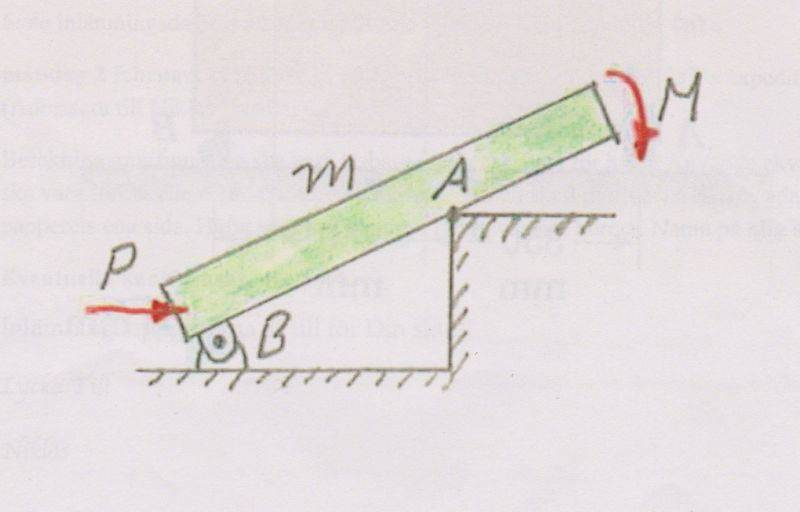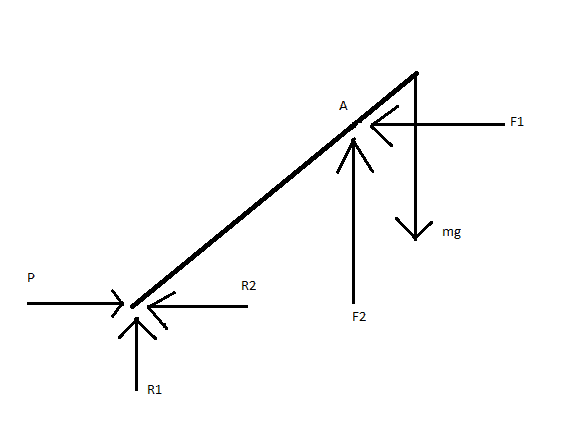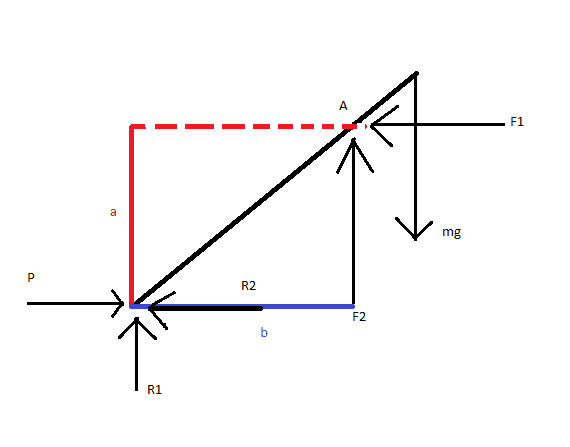# Yet another statics problem involving several forces

• mechanim
In summary, the body is connected to the ground with a movable joint at B and slides without friction at A. The forces acting on the body include P, R1, R2, F1, and F2, with P and M not specified. The equations to be used are ΣF=0, ΣFx=0, ΣFy=0, and ΣM=0. The calculations involve finding the forces acting on the body and simplifying the drawing.

## Homework Statement

The body is connected to the ground with a moving joint at B, the body slides with no friction at A.## Homework Equations

ΣF=0 => ΣFx=0 & ΣFy=0
ΣM=0

## The Attempt at a SolutionAt this point I started calculating:
ΣFx=>P-R2-F1, so: P=R2+F1
ΣFy=> R1+F2-mg=0, so: R1+F2=mg

I'm quite confused when it comes to the moment around B, I know that Moment=force*distance, but how do I draw all the distance that I need?

I thought about something like that:So, the distance between F1 and the point B is a, while the distance between F2 and B is b.
Knowing this, I assume that the moment would look like that:

Mb: P+mg-F2*b-F1*a-R2-R1=0
now I replace P and mg according to the previous equations:
Mb: R2+F1+R1+F2-F2*b-F1*a-R2-R1=0
now the weird part is that I could reduce almost everything in the equation above, so that I would only be left with:
Mb: b-a=0
but it doesn't make any sense.

I would appreciate any help, thank you in advance!

Two things:
1) the 'mg' force always acts from the center of mass of the object
2) how exactly does the body slide? Seems to be some mistake there

•mechanim
2) how exactly does the body slide? Seems to be some mistake there

I don't think they are saying it slides. I think the point is that there is no friction at "a" so that it could slide if "b" wasn't there. If there was friction at "a" (or if "a" was another mounting like that at "b") then I think you would find it hard to calculate how forces are shared between "a" and "b".

•mechanim
Deleted.

It says exactly that the body slides at A with no friction. I don't know exactly what they mean by that either. What do you think about the equations though?

What
mechanim said:
It says exactly that the body slides at A with no friction. I don't know exactly what they mean by that either. What do you think about the equations though?
what does it say about P and M?? In fact, what is the question as exactly worded?

That is the problem, it doesn't say anything about either P or M. I assume that M is supposed to be Moment, while P is a force, but the whole question is "The body in the picture is connected to the ground with a movable joint and slides without friction at A. Simplify the drawing and find the forces". It doesn't even say how far in the calculations I'm supposed to go.